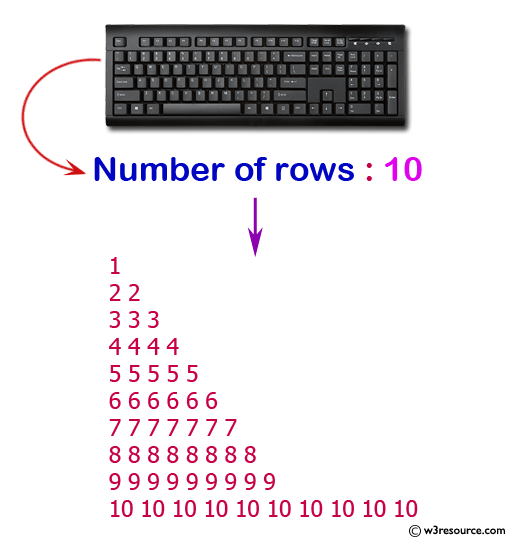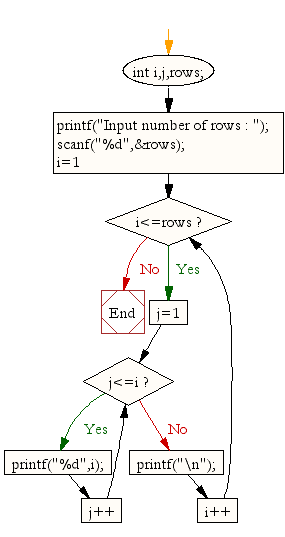﻿ C : Right angle triangle pattern, repeat a number in a row

# C Exercises: Display the pattern like right angle triangle which repeat a number in a row

## C For Loop: Exercise-11 with Solution

Write a program in C to make such a pattern like a right angle triangle with a number which will repeat a number in a row.
The pattern is as follows:

``` 1
22
333
4444
```

Visual Presentation:Sample Solution:

C Code:

``````#include <stdio.h>  // Include the standard input/output header file.

int main() {
int i, j, rows;  // Declare variables 'i' and 'j' for loop counters, 'rows' for user input.

printf("Input number of rows : ");  // Print a message to prompt user input.
scanf("%d", &rows);  // Read the value of 'rows' from the user.

for (i = 1; i <= rows; i++) {  // Start a loop to generate rows.
for (j = 1; j <= i; j++)  // Nested loop to print numbers based on the current row.
printf("%d", i);  // Print the value of 'i'.

printf("\n");  // Move to the next line for the next row.
}
return 0;
}
```
```

Sample Output:

```Input number of rows : 10
1
22
333
4444
55555
666666
7777777
88888888
999999999
10101010101010101010
```

Explanation:

```for (i = 1; i <= rows; i++) {
for (j = 1; j <= i; j++)
printf("%d", i);
printf("\n");
}
```

In the said for loop, the variable i is initialized to 1, and the loop will continue as long as i is less than or equal to the value of variable 'rows'. In each iteration of the outer loop, another loop is started with variable j, initialized to 1, and it will continue as long as j is less than or equal to the value of i.

In each iteration of the inner loop, the printf() function will print the value of j to the console. This value will be printed j times, as the value of j is increasing by 1 in each iteration of the inner loop.

After the inner loop completes, the outer loop will print a newline character (\n) to create a new line.

The outer loop will increment the value of i by 1, and the process will repeat until the condition i<=rows is no longer true.

Flowchart:C Programming Code Editor:

What is the difficulty level of this exercise?

Test your Programming skills with w3resource's quiz.

﻿